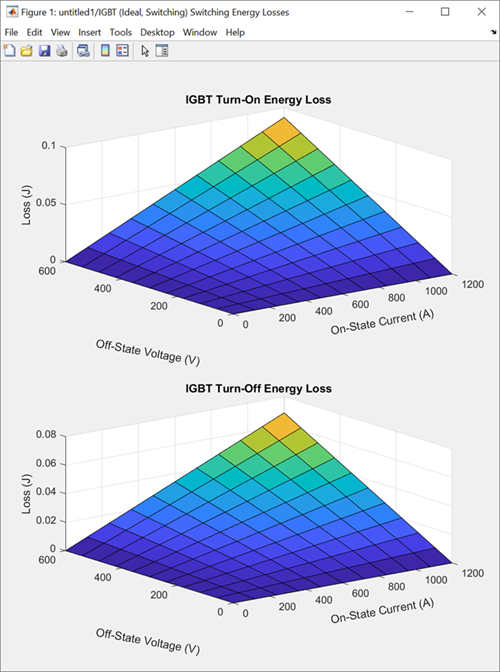## Plot Basic Characteristics for Semiconductor Blocks

You can plot the basic I-V characteristics for semiconductor blocks based on the current block parameter values without simulating a model.

To plot the basic characteristics, right-click a semiconductor block in your model that supports this option and select Electrical > Basic characteristics from the context menu. The software computes a set of bias conditions using the block parameter values and then opens a figure window containing a plot of the DC I-V characteristics for the block. For example, the Basic characteristics option generates this figure for the N-Channel MOSFET block with default parameter values.If you change the block parameter values and plot the characteristics again, the plot opens in a new window. You can compare the plots side-by-side to see how the parameter values affect the I-V characteristics. For example, if you set the Gate-source voltage, Vgs, for R_DS(on) parameter of the N-Channel MOSFET block to `20` `V`, and select the Basic characteristics option again, you generate this plot.You can use the Basic characteristics option with these semiconductor blocks.

Block Modeling option Parameter Values
Diode`No thermal port`
IGBT (Ideal, Switching)`No thermal port` or ```Show thermal port```
MOSFET (Ideal, Switching)`No thermal port` or ```Show thermal port```
N-Channel IGBT ```Full I-V and capacitance characteristics | No thermal port```
N-Channel MOSFET`Threshold based` or ```Surface potential based```
P-Channel MOSFET`Threshold based` or ```Surface potential based```
N-Channel LDMOS FET`No thermal port`
P-Channel LDMOS FET`No thermal port`
N-Channel JFET`No thermal port`
P-Channel JFET`No thermal port`
NPN Bipolar Transistor`No thermal port`
PNP Bipolar Transistor`No thermal port`
Light-Emitting Diode`No thermal port`
Photodiode`No thermal port`

Note

The Basic characteristics option has additional capabilities if you use the option with the IGBT (Ideal, Switching) or MOSFET (Ideal, Switching) blocks. For more information, see Plot Basic Characteristics for Ideal Semiconductors.

The N-Channel MOSFET and P-Channel MOSFET blocks also support the Explore characteristics option. Use this option to perform an in-depth study of block characteristics and match the block behavior to a set of target characteristics. To enable this option, set the Modeling option parameter of the block to `Surface potential based`. Then, right-click the block and select Electrical > Explore characteristics from the context menu. For more information about this option, see MOSFET Characteristics Viewer.

### Plot Basic Characteristics for Ideal Semiconductors

Since R2023b

The Basic characteristics option has additional capabilities with these blocks from the Semiconductors library:

The plots you generate with the Basic characteristics option depend on the values you specify for the Modeling option, On-state behavior and losses, and Integral protection diode parameters of the block.

To plot only the on-state and off-state I-V characteristics of the switching device, set the Modeling option parameter to ```No thermal port``` and set the Integral protection diode parameter to `None`. Then, right-click the block in your model and select Electrical > Basic characteristics from the context menu. For example, the Basic characteristics option generates this plot for the IGBT (Ideal, Switching) block with default parameter values.You can also plot the on-state and off-state I-V characteristics of an integral protection diode. Set the Integral Protection Diode parameter to `Diode with no dynamics` or ```Diode with charge dynamics```. The Basic characteristics option plots the I-V characteristics of the switching device and the integral protection diode side-by-side.You can also generate surface plots of the turn-on energy loss and turn-off energy loss as functions of the on-state current and off-state voltage. Set the Modeling option parameter to ```Show thermal port``` and set the On-state behavior and losses parameter to `Specify constant values`. The Basic characteristics option opens the surface plots in this figure in a separate window to the I-V characteristics plots.If you set the On-state behavior and losses parameter to `Tabulate`, the Basic characteristics option plots the results over a range of temperatures. Surface plots for different temperatures open in different windows. The I-V characteristics plots show the results for different temperatures on a single graph in an additional window.

### Plot Basic Characteristics of Semiconductor Sensors

These semiconductor sensor blocks in the Sensors & Transducers library support the Basic characteristics option if you disable the thermal port of the block.

• Light-Emitting Diode — The Basic characteristics option plots the I-V characteristics and current versus optical power.

• Photodiode — The Basic characteristics option plots the I-V characteristics at different flux densities.

To disable the thermal port of the block, set the Modeling option parameter to `No thermal port`.